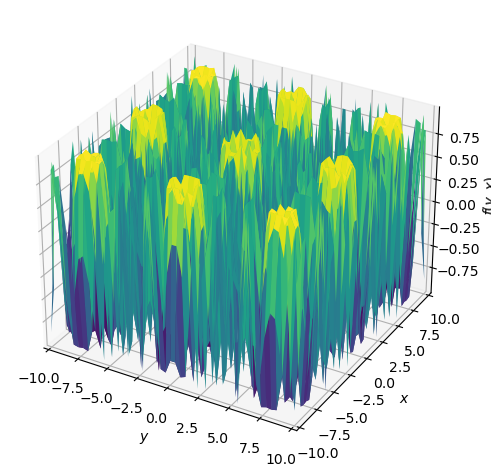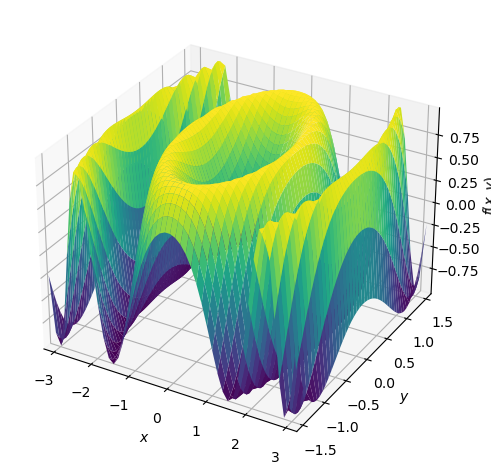# How to graph a two-variable function as a surface (in Python, using SymPy)

See all solutions.

Assume we have a mathematical formula in the variables $x$ and $y$ and we would like to plot a graph of it using a 3D coordinate system.

## Solution

This answer assumes you have imported SymPy as follows.

1
2
from sympy import *                   # load all math functions
init_printing( use_latex='mathjax' )  # use pretty math output


First, we need a two-variable function that we wish to plot.

1
2
3
var( 'x y' )
formula = sin( x**2 + y**2 )
formula


$\displaystyle \sin{\left(x^{2} + y^{2} \right)}$

You can use plot3d, but you have to import it specifically, because it is not imported by default with the rest of SymPy.

1
2
from sympy.plotting.plot import plot3d
plot3d( formula )1
<sympy.plotting.plot.Plot at 0x7f085354fa60>


Specify the minimum and maximum values for both $x$ and $y$ as follows. In this example, I keep $-\pi\leq x\leq\pi$ and $-\frac\pi2\leq y\leq\frac\pi2$.

1
plot3d( formula, (x,-pi,pi), (y,-pi/2,pi/2) )1
<sympy.plotting.plot.Plot at 0x7f07e5878a60>